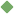decimal 1. [ noun ] (mathematics) a proper fraction whose denominator is a power of 10 Synonyms: decimal_fraction Related terms: proper_fraction circulating_decimal decimalise To share this definition press "text" (Facebook, Twitter) or "link" (blog, mail) then paste text link 2. [ adjective ] divided by tens or hundreds Examples: "a decimal fraction" "decimal coinage" Related terms: quantitative 3. [ adjective ] numbered or proceeding by tens; based on ten Synonyms: denary Examples: "the decimal system" Related terms: quantitative 4. [ noun ] (mathematics) a number in the decimal system Related terms: number decimalize Similar spelling: decimalize   decimalise